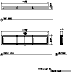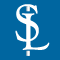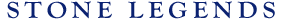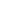HOMEPRODUCTSIDEA CENTERSERVICESTECHNICALABOUT USHello Guest Login  |  Become Member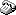800-398-1199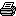214-398-1293MapEmail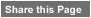Click to Share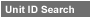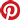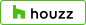Select which variables you would like to search by.Type DescriptionPrimary ViewPrimary ModifierUnit NameView Definitions

View Definitions

View DefinitionsX-Dim Y-Dim Z-Dim XX-Dim YY-Dim ZZ-Dim R-Dim D-Dim P-Dim
 Click column heading to re-sort results list.Product Name Primary View Modifier Pri Dimx  y  z Alt Dimxx  yy  zz Alt2 Dimr  d  p E\$timated Thumbnail Burton Signage Circular X = 16" Y = 16"Z = 2-3/4" XX = n/a YY = n/aZZ = n/a R = 8" D = 16"P = n/a \$ 80.00Carissa Signage Rectangular X = 23-5/8" Y = 38-5/8"Z = 4-5/8" XX = n/a YY = n/aZZ = n/a R = n/a D = n/aP = n/a \$ 100.00Carter Signage RadiusWall X = 33-3/4" Y = 214"Z = 30-1/2" XX = n/a YY = n/aZZ = n/a R = 233" D = n/aP = n/a \$ 576.00Chance Signage Rectangular X = 31-1/2" Y = 56-1/2"Z = 3-7/8" XX = n/a YY = n/aZZ = n/a R = n/a D = n/aP = n/a \$ 145.00Chanda Signage Circular X = 24" Y = 24"Z = 4-1/8" XX = n/a YY = n/aZZ = n/a R = 10" D = 24"P = n/a \$ 100.00Charleston Signage Rectangular X = 35-3/8" Y = 172-1/4"Z = 3" XX = n/a YY = n/aZZ = n/a R = n/a D = n/aP = n/a \$ 525.00Chelsea Signage Rectangular X = 31" Y = 99-1/8"Z = 6-3/8" XX = n/a YY = n/aZZ = n/a R = n/a D = n/aP = n/a \$ 279.00Enya1 Signage Circular X = 22" Y = 22"Z = 4" XX = 36" YY = 36"ZZ = 1-1/2" R = n/a D = 36"P = n/a \$ 165.00Fire&Rescue Signage Circular X = 16" Y = n/aZ = 4-9/16" XX = 16" YY = n/aZZ = n/a R = n/a D = n/aP = n/a \$ 375.00Flatface3 Signage X = 46" Y = 46"Z = 3" XX = n/a YY = n/aZZ = n/a R = n/a D = n/aP = n/a \$ 115.00FlatfaceRecessed Signage Rectangular X = 30" Y = 60"Z = 4" XX = 22" YY = 50"ZZ = 3" R = n/a D = n/aP = n/a \$ 144.00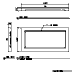Lamen 1 Signage Rectangular X = 20" Y = 20"Z = 4" XX = n/a YY = n/aZZ = n/a R = n/a D = n/aP = n/a \$ 120.00Lily 1 Signage Rectangular X = 27" Y = 24"Z = 3" XX = n/a YY = n/aZZ = n/a R = 27" D = n/aP = n/a \$ 240.00Lily 2 Signage Rectangular X = 20" Y = 18"Z = 3" XX = n/a YY = n/aZZ = n/a R = 20" D = n/aP = n/a \$ 185.00Onora Signage Circular X = 33-1/4" Y = 33-1/4"Z = 5" XX = n/a YY = n/aZZ = 4" R = n/a D = 33-1/4"P = n/a \$ 224.99Phoenix Oval Signage Elliptical Art X = 45" Y = 30"Z = 6" XX = n/a YY = n/aZZ = n/a R = n/a D = n/aP = n/a \$ 575.00Recessed Signage Rectangular X = 16" Y = 76-7/16"Z = 4" XX = 12" YY = 72-7/16"ZZ = 3" R = n/a D = n/aP = n/a \$ 104.00